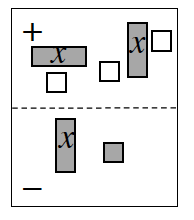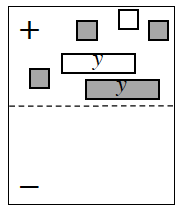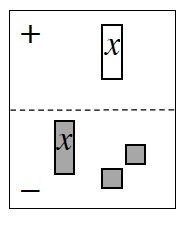### Home > AC > Chapter 2 > Lesson 2.1.4 > Problem2-44

2-44.

Write and simplify the algebraic expression shown in each expression mat below.1.$\left(2x − 3\right) − \left( ? \right)$

1.Which tile pairs make zeros?

1.$−x −\left(x + 2\right) = −2x − 2$

Click the link at right for the full version of the eTool: 2-44a HW eTool